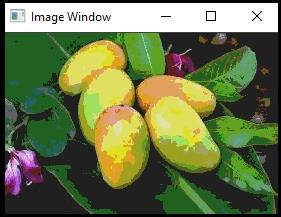# How to reduce the color using Iterator Method in OpenCV?

OpenCV has C++ STL compatible 'Mat iterator' class. Using this 'Mat iterator' class, we can access pixels very easily. We have to create an object of 'Mat iterator' class. We can do it as 'Mat_<Vec3b>: : iterator example'. We have to use an underscore after 'Mat' like 'Mat_' because it is a template method. In this method, the return type must be specified while creating an object of 'iterator' class. That is why we have declared the datatype <Vec3b>.

The following program demonstrates how to reduce the color using Iterator Method in OpenCV.

## Example

#include<iostream>
#include<opencv2/highgui/highgui.hpp>
using namespace std;//Declaring std namespace
using namespace cv;//Declaring cv namespace
void reducing_Color(Mat& image, int div = 64){ //Declaring the function//
Mat_<Vec3b>::iterator iterator_start;//Declaring starting iterator//
iterator_start = image.begin<Vec3b>();//Obtain iterator at initial position//
Mat_<Vec3b>::iterator iterator_end;//Declaring ending iterator//
iterator_end = image.end<Vec3b>();//Obtain iterator an end position//
for (; iterator_start != iterator_end; iterator_start++){ //Loop for all pixels//
(*iterator_start) = (*iterator_start) / div * div + div / 2;//Process pixels of first channel//
(*iterator_start) = (*iterator_start) / div * div + div / 2;//Process pixels of second channel//
(*iterator_start) = (*iterator_start) / div * div + div / 2;//Process pixels of third channel//
}
}
int main() {
Mat image;//taking an image matrix//
}